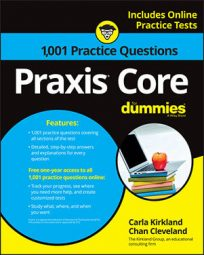##### Praxis Core: 1,001 Practice Questions For DummiesFractions and decimals don't work well together. This means that if you run into any questions on the Praxis Core exam involving fractions and decimals, you'll probably have to start by doing some conversions.

The first practice question starts off with a simple conversion of a fraction to a decimal, while the second question asks you to add a fraction to a decimal.

## Practice questions

1. What is

in decimal form?

A. –2.125 B. –2.25 C. –2.1 D. –2.8 E. –2.012

2. What is the sum of 1/12 and 3.178, rounded to the nearest thousandth?

A. 3.3 B. 3.26 C. 3.262 D. 3.026 E. 3.261

1. The correct answer is Choice (A).

First, put the mixed number in improper fraction form:

Next, convert the fraction to a decimal by dividing the numerator by the denominator:

2. The correct answer is Choice (E).

The answer choices are in decimal form, so put 1/12 in decimal form by dividing 1 by 12:

The quotient has 3 repeated infinitely after the decimal point. When that happens, you can round to the nearest ten-thousandth (four places after the decimal point) to make reliable calculations for the Praxis Core exam:

Now you can add 8.3333 to 3.178, which is already in decimal form:

The question asks for the sum rounded to the nearest thousandth, which is three places past the decimal point. Because the fourth digit after the decimal point is 3, round down by dropping the last 3 and keeping the 1: